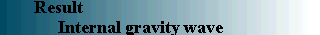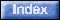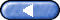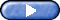When the amplitude of wave is sufficiently large, mean flow occurs. Figure 7 shows the propagation of the internal gravity wave, when the mean flow is rightward at the top. It is clear that the leftward-propagated wave is absorbed by the mean flow and only the rightward-propagated wave is visible in contrast to the case of no mean flow. This absorption of the wave occurs at a level where the velocity of the mean flow is equal to that of the phase velocity of the wave. This is called the critical level. Especially, the level where the phase velocity of the wave is equal to the velocity of the fluid is called the critical level. From Figure 7, it is clearly seen that the wave in the same direction with the mean flow is absorbed, and only the leftward wave propagating in the opposite direction prevails. Also, the critical level does not exist in the mean flow. It should also be noted that the mean flow creates the oscillation. The pattern of the internal gravity wave in this case is visualized in Figure 8. The pattern of the wave changes with the change in mean flow.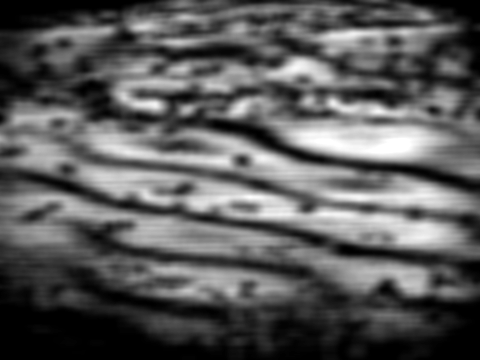Figure 7 Internal gravity wave in shear flow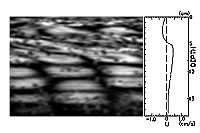Figure 8 Internal gravity wave distorted by mean flow